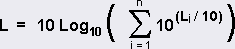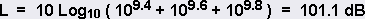USA ▼
Calculators > Decibel Calculator

# Decibel Calculator

The NoiseMeters decibel calculator can be used to carry out addition and subtraction of dB values between 0 and 200 dB.

## dB Calculator

0
dB

### Decibel Addition and Subtraction

Sound levels are generally expressed in decibels, which are logarithmic and so cannot be manipulated without being converted back to a linear scale. You must first antilog each number, add or subtract and then log them again in the following way:For example, adding three levels 94.0 + 96.0 + 98.0:Or use the calculator!